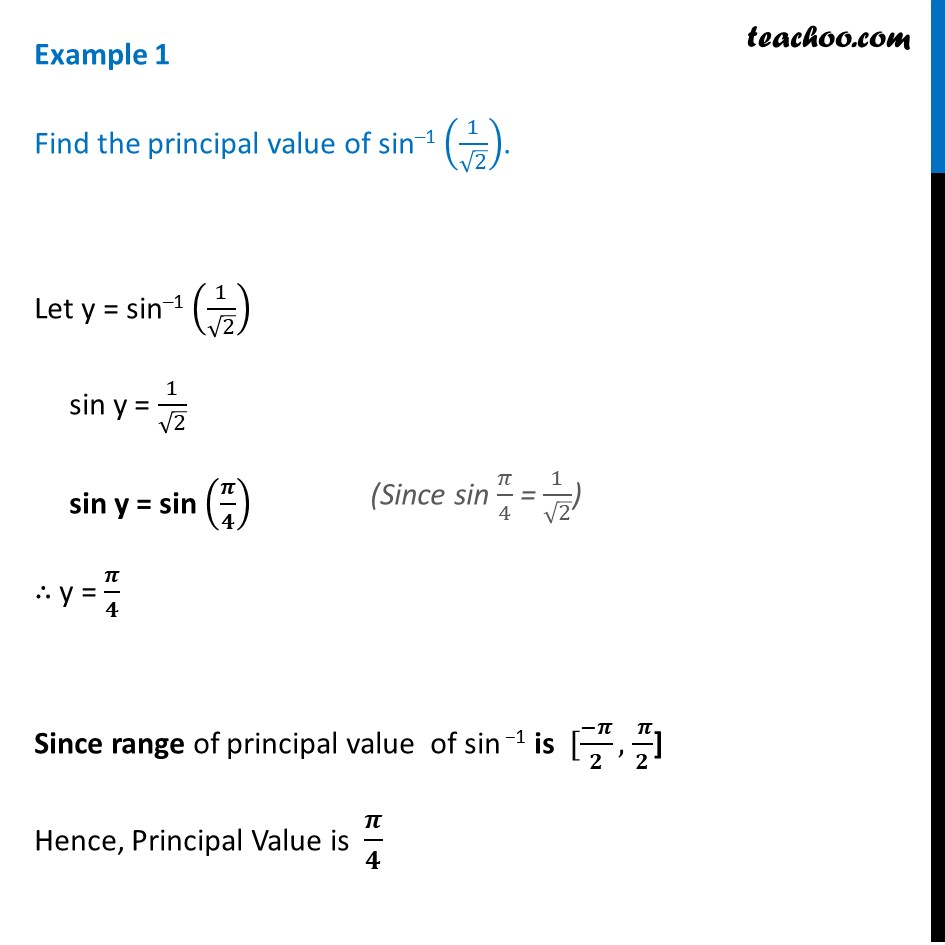Examples

Chapter 2 Class 12 Inverse Trigonometric Functions
Serial order wiseLearn in your speed, with individual attention - Teachoo Maths 1-on-1 Class

### Transcript

Example 1 Find the principal value of sin–1 (1/√2). Let y = sin–1 (1/√2) sin y = 1/√2 sin y = sin (𝝅/𝟒) ∴ y = 𝝅/𝟒 Since range of principal value of sin −1 is [(−𝝅)/𝟐, ( 𝝅)/𝟐] Hence, Principal Value is 𝝅/𝟒